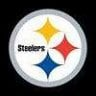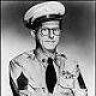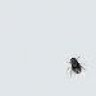# Odds of inside straight.

R

##### Guest
I hope this isn't too stupid of a question but I am a real novice.

If there are three players left at the table and the flop would be filled with an inside straight is it reasonable to assume there is about a 50% chance someone will have the card to fill it?#### Jack Daniels

##### Charcoal Mellowed
Not sure I'm really following the question (maybe others are and it is just me). But you wouldn't have an inside straight on board until the turn came out. So is that what you mean? Once the turn is out, what is the % chance that someone is holding one of the gutshot cards? And is it just three people left at the table or just three in the hand?

Sorry if I'm seeming nit picky, it's late and I should have gone to bed two hours ago.#### Effexor

##### Cardschat Elite
I didn't understand the question either.

R

##### Guest
Hi, Yea -sorry i didn't make myself clear. 3 in the hand and you are waiting for the river card.#### Bombjack

##### Legend
It depends what the card is. People play more higher cards than low cards. Also your question is not clear. Are you one of the three players, and so know that you don't have it, or are you talking about three other players whose cards you don't know?

You can get an estimate of the probability by assuming people are playing random cards. As with most problems like this, to work out the probability of at least 1 card of a particular type being out there, you do it by working out the probability of there being none, and subtract this from 1. To work out the probability of there being none, you take the probability of each card NOT being the required rank and multiply all of these together.

I'll leave it as an exercise for you to work this out. If you post your answer with working and I'll tell you if it's right.#### bubbasbestbabe

##### Suckout Queen
Let's clarify the question. On the board is a 3, 4, 5, and 7. You have 3 players at the table,(including yourself). And you want to know what the odds are for one of the three players having the gutshot card?

I stink at math but I can help you with the question itself. I also would be interested in the answer.

R

##### Guest
Clarification

Yep - that is the question precisely.#### Welly

##### Guest
This isnt the correct way for you to approach your problem. You should be more worried about your own card holding and then assigning cards to the others based on their betting of this hand, and their previous behaviour.

Eg to oversimplify it :-

If a player launches at the turn, and you have seen him to be a player that very rarely bluffs then you should be more concerned about the cost to you of calling, versus the probability of you outdrawing him.

If a player launches at the turn and you have seen that he bluffs situations like this a lot then you can split the odds in 2. Half for the bluff, half that he holds it, and again look at the cost of calling vs the average probabilty of outdrawing him.

edit...ps, if you post the hand history we can help you with the pot odds and whether you have positive equity

Last edited:#### Bombjack

##### Legend
OK if there are 3 players including yourself, that means there are 2 other players, holding a total of 4 cards. You don't hold a 6 yourself. What are the chances that at least one of these 4 cards is a 6? Answer these questions:

a) What is the chance of the first of these NOT being a 6? (Hint: how many cards of the 52 are there remaining that you haven't seen? How many of these are NOT a 6?)

b) Given that the first card is not a 6, what's the chance of the second of these NOT being a 6? (Hint: number of cards remaining is now 1 less, as is the number of cards that is not a 6.)

c) Repeat for 3rd and 4th card.

d) Chance of ALL 4 cards NOT being a 6 is these chances multiplied together.#### Jack Daniels

##### Charcoal Mellowed
Well, the philisophical answer is 50/50. They either have it or they don't.And, unfortunately for you question, there is no absolute way of calculating the probability of one of them holding the correct card (short of the valiant attempt made by Bombjack above).
But even this calculation is skewed because it focuses on the pure randomness of the cards and can't consider playable hands. This is why poker is a game of incomplete information. If there was a realistic way to do this accurately, we'd never make bad calls.

I like Welly's idea of posting the HH for us to look at.#### robwhufc

##### Cardschat Elite
If there are three players left at the table and the flop would be filled with an inside straight is it reasonable to assume there is about a 50% chance someone will have the card to fill it?
Nope, depends on the card. 3 remaining players will have 6 cards - If the card is a king, there is a far higher chance that this is being held post flop than if the card is a 6.#### Dorkus Malorkus

##### HELLO INTERNET
2 other players (I'm assuming the three players left includes you, if only to avoid having to extend the following working even further), they hold 4 'mystery' cards.

There are four 'gutshot' cards to choose from remaining deck of 46 (52, less 4 board cards, less your two cards)

Let's call the cards in villain's hands A,B,C, and D, and assume we're working on a completely 'random hand' basis.

P(A is a 6) = 4/46 = 0.087
P(A is not a 6) = 1-0.087 = 0.913

| = "given that", for those who aren't acquainted with stat notation.P(B is a 6 and A is not a 6) = 0.913*(4/45) = 0.913*0.089 = 0.081
P(B is a 6 | A is not a 6) = P(B is a 6 and A is not a 6) / P(A is not a 6)
P(B is a 6 | A is not a 6) = 0.081 / 0.913
P(B is a 6 | A is not a 6) = 0.089

P(A and B are not 6s) = 0.913*(1-0.089) = 0.831
P(C is a 6 and A and B are not 6s) = 0.831*(4/44) = 0.831*0.091 = 0.076
P(C is a 6 | A and B are not 6s) = P(C is a 6 and A and B are not 6s) / P (A and B are not 6s)
P(C is a 6 | A and B are not 6s) = 0.076 / 0.831
P(C is a 6 | A and B are not 6s) = 0.092

P(A, B, and C are not 6s) = 0.831*(1-0.092) = 0.755
P(D is a 6 and A, B, and C are not 6s) = 0.755*(4/43) = 0.755*0.093 = 0.070
P(D is a 6 | A, B, and C are not 6s) = P(D is a 6 and A, B, and C are not 6s) / P(A, B, and C are not 6s)
P(D is a 6 | A, B, and C are not 6s) = 0.070 / 0.755
P(D is a 6 | A, B, and C are not 6s) = 0.093

P(A, B, C, or D are a 6) = P(A is a 6) + P(B is a 6 | A is not a 6) + P(C is a 6 | A and B are not 6s) + P(D is a 6 | A, B, and C are not 6s)
P(A, B, C, or D are a 6) = 0.087 + 0.089 + 0.092 + 0.093
P(A, B, C, or D are a 6) = 0.361 = 36%

This doesn't account for situations where more than one of A,B,C,or D are a 6, but the probability of that is low and won't affect the outcome much. I've just realised after working all this I could have accounted for it by working Bombjack's way, but I'm too lazy to go through it again. I wouldn't think it would change the result more than a couple of percent though.

I might have gone wrong somewhere (it has been a few years since I did my maths course, after all!), but the result is around about what I expected, so perhaps not.#### Bombjack

##### Legend
OK seeing as the response has been non-existent, I'll answer my own questions:
a) 43/47
b) 42/46
c) 41/45, 40/44
d) Chance of all not the straight card is 43/47*42/46*41/45*40/44 = 69.2%. So chance of someone holding it is 30.8%.

As has been pointed out, this is only an average, and it depends what the card is, if it's high or low, although this is pretty impossible to quantify without knowing a list of your opponents' starting hands.#### Dorkus Malorkus

##### HELLO INTERNET
OK seeing as the response has been non-existent, I'll answer my own questions:
a) 42/46
b) 41/45
c) 40/44, 39/43
d) Chance of all not the straight card is 42/46*41/45*40/44*39/43 = 68.5%. So chance of someone holding it is 31.5%.

As has been pointed out, this is only an average, and it depends what the card is, if it's high or low, although this is pretty impossible to quantify without knowing a list of your opponents' starting hands.

FYP, I think.

I dunno where you get the 43/47 and numbers following on from that, perhaps you're forgetting the turn card is out?I now tiredly wonder why my figure is higher than yours when it should be around a couple of percent lower. Meh.

lolol i just noticed i did about 4528678 times more working than needed. Maths degrees do stupid things to your mind and make you forget simple ways of doing stuff... sigh.

Last edited:#### Bombjack

##### Legend
Doh, he said in his first post the "flop would be filled by an inside straight". Which doesn't make any sense. OK, for the TURN the sum is: 1-42/46*41/45*40/44*39/43 = 31.4%

Edit: just noticed Dorkus did this in editing my post above.

Poker Odds - Pot & Implied Odds - Odds Calculator